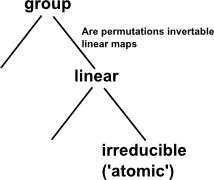# Maths - Group Theory - Linear Group Types

## Represention Theory

Represention theory is a specialisation of group theory that is restricted to vector spaces.## Classification of Groups## Conjugacy Classes

There is a 1:1 correspondence between transitive actions and conjugate classes.

 Conjugacy classes of subgroups Transitive actions of G collection of subgroups conjugate to some given subgroup. Transitive = one orbit Any action = sequence of transitive actions.

## Actions

We can look at the concepts that we used for permutations, now that we are restricting ourselves to linear maps:

### Stabiliser

Subgroup of G consisting of all elements h such that:

h x = x

h is the stabiliser of x

## Mat(n,F)

May be any n×n matrix containing R, C, H or O elements.

• Mat(n, R)
• Mat(n,C)
• Mat(n,H)
• Mat(n, O)

The definitions don't make it totally clear whether Mat(2,C) is any 2×2 matrix whose elements are C or is it the matrix algebra over the centre of C
From Wikipedia: "The term center or centre is used in various contexts in
abstract algebra to denote the set of all those elements that commute with
all other elements".

## SL(n, F) The Special Linear Group

Consisting of n×n matrices where each element is of type 'F' with determinant =1.Symmetry and the Monster - This is a popular science type book which traces the history leading up to the discovery of the largest symmetry groups.